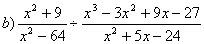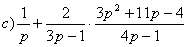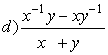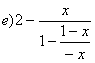KING FAHD UNIVERSITY OF PETROLUEM AND MINERALS

Faculty of Science – Math Prep-Year Program

Math 001 - Term 031

P.1:

Q1) Which of the following statement is TRUE and which is FALSE .State the

reason :

a)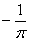is the multiplicative inverse of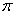.

b)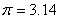.

c) 0, 1, 2, 3, 4, … are positive integers .

d) Every integer is either prime or composite .

e) Every rational number has a multiplicative inverse .

f) The set of irrational numbers is not closed under addition and multiplication .

g) 1 is the only positive integer that is not prime nor composite

h) 1.7 +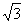is a rational number .

i) The set { -1,0,1} is closed under addition .

j)The set { -1,0,1} is closed under multiplication .

k) 51 is a prime number

l) every prime number is and odd number .

Q2)  Name the property of real number or the property of equality of the following :

1)      (a + b ) + c = c + ( a + b )

2)      (4.x).y = y.(4.x)

3)      (4.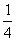)z = 1.z

4)      3 + (-3) = 0

5)      1.z = 1.z

6)      3(x+4) = 3x+12

7)      a(bc) = a(bc)

Q3) If  A=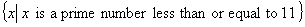B=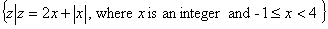,

1-      List all elements of A and B

2-      Find A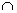B

P.2:

Q1) Which of the following statement is TRUE and which is FALSE .State the

reason :

a) If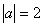and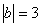, then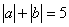.

b)  The distance between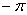and -3 is  is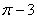.

c)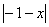=.

d) The distance between two points in real in the real line is always positive .

e)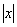is positive.

f) If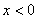, then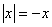.

j) If, then.

h) If, then.

k).

Q2)  Write the following expressions without the absolute value notation :

1-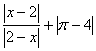2-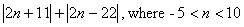3-Q5 Given the inequalityor,

1-      Graph the given inequality on a real line .

2-      Write the given inequality as an interval notation .

P.3:

Q1) Simplify Each of the following expressions:b.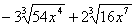Q2) Find the value of each of the following :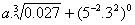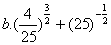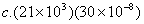. Write the answer in scientific notation.

Q3) Rationalize the denominator in the following :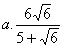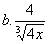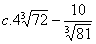P.4:

Q1) Given the polynomial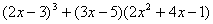a. Write this polynomial in the standard form .

b. Complete the following table :

 The leading coefficient is The constant term is The coefficient of x is

Q2) Which of the following is a polynomial :

a.b. 5                  c.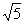d.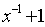e.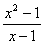f.g.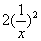h.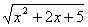i.j.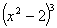k.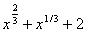P.5:

Q1) Factor each of the following completely :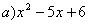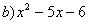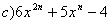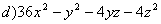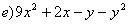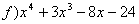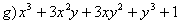Q2) Find all positive values of k such thatis a perfect-square

trinomial.

P.6:

Simplify :